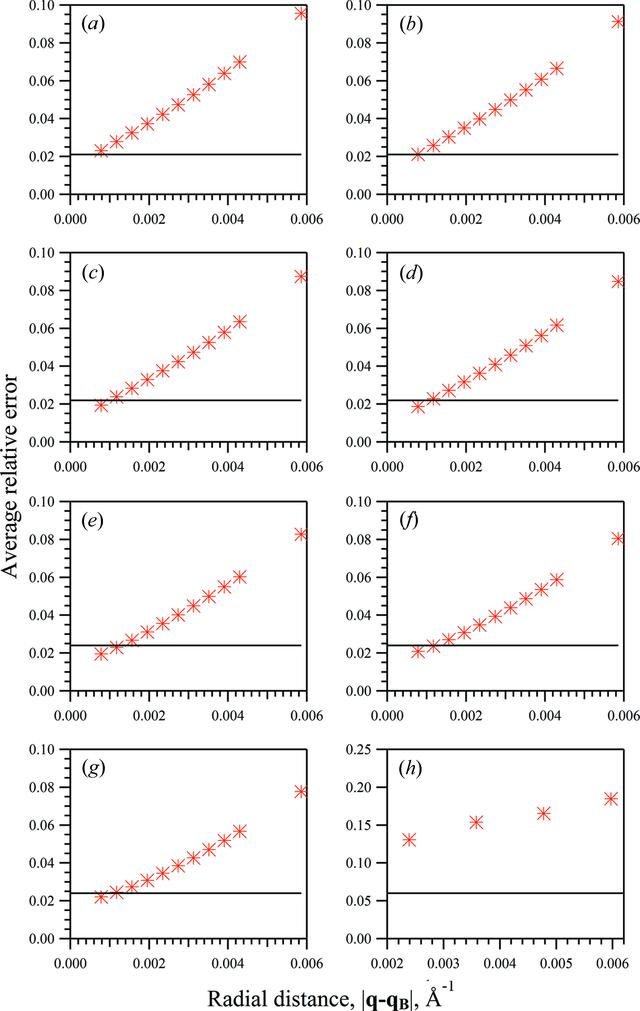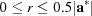disable zoom     view article Figure 1 Structure-factor moduli average relative errors from the two-dimensional whole-pattern fitting approach (line) and from the integration approach (* symbol) against integration area for merged diffraction patterns from sets of 2000 crystallites with varying mean unit-cell dimensions along the Y direction, 〈NY〉, and with a mean of 10 unit cells along the X direction, 〈NX〉: (a) 〈NY〉 = 30, (b) 〈NY〉 = 35, (c) 〈NY〉 = 40, (d) 〈NY〉 = 45, (e) 〈NY〉 = 50, (f) 〈NY〉 = 55, (g) 〈NY〉 = 60, (h) 〈NY〉 = 30. (a)–(g) The diffraction patterns were simulated on a 1024 × 1024 pixel array to 5 Å resolution. (h) The diffraction pattern was simulated on a 340 × 340 pixel array to 5 Å resolution. The integration area was defined by a circle with radius varied in the range.

IUCrJ
Volume 3| Part 2| March 2016| Pages 127-138
ISSN: 2052-2525
Follow IUCrJ